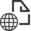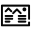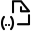ReleasedDataset

# SGG-UGM-1: the high resolution gravity field model based on the EGM2008 derived gravity anomalies and the SGG and SST data of GOCE satellite

## Cite as:

Liang, Wei (2018): SGG-UGM-1: the high resolution gravity field model based on the EGM2008 derived gravity anomalies and the SGG and SST data of GOCE satellite. GFZ Data Services. http://doi.org/10.5880/icgem.2018.001

## Status

I   N       R   E   V   I   E   W : Liang, Wei (2018): SGG-UGM-1: the high resolution gravity field model based on the EGM2008 derived gravity anomalies and the SGG and SST data of GOCE satellite. GFZ Data Services. http://doi.org/10.5880/icgem.2018.001

##Abstract

SGG-UGM-1 is a static gravity field model based on EGM2008 derived gravity anomalies and GOCE Satellite Gravity Gradiometry (SGG) data and the Satellite-to-Satellite Tracking (SST) observations up to degree and order 2159. Block-diagonal normal equation system up to degree and order 2159 are formed with EGM2008 gravity anomaly data using block-diagonal least squares method. Fully occupied normal equation system up to degree and order 220 are formed by GOCE SGG data and the SST observations along the GOCE orbit based on least-squares analysis. The diagonal components (Vxx, Vyy, Vzz) of the gravitational gradient tensor are used to form the system of observation equations with the band-pass ARMA filter. The point-wise acceleration observations (ax, ay, az) along the orbit are used to form the system of observation equations up to the maximum spherical harmonic degree/order 130. SGG-UGM-1 is resolved by combination of the two normal equation systems using least squares method.

It is the first generation of high-resolution gravity model in ICGEM developed by School of Geodesy and Geomatics (SGG), Wuhan University (WHU). More details about the determination of the model are given in our paper “The determination of an ultra high gravity field model SGG-UGM-1 by combining EGM2008 gravity anomaly and GOCE observation data” (Liang W, Xu X, Li J, et al. Acta Geodaeticaet Cartographica Sinica. 2018, 47(4): 425-434. DOI:10.11947/j. AGCS.2018.20170269) and “A GOCE only gravity model GOSG01S and the validation of GOCE related satellite gravity models ” (Xu X, Zhao Y, Reubelt T, et al. Geodesy and Geodynamics. 2017, 8(4): 260-272. http://dx.doi.org/10.1016/j.geog.2017.03.013). The work is supported by the Natural Science Foundation of China (Nos. 41774020, 41210006 and 41404020

## Methods

SGG-UGM-1 is a global gravity field model computed by the combination of EGM2008 gravity anomalies and GOCE SGG,SST data

Input data:
-- EGM2008 derived gravity anomalies (5' x 5' resolution)
-- GOCE SGG data: EGG_NOM_2 (GGT: Vxx, Vyy, Vzz) in GRF
-- GOCE SST data: SST_PKI_2, SST_PCV_2, SST_PRD_2

Processing procedure:
-- Block-diagonal normal equation system up to degree and order 2159 are formed with EGM2008 gravity anomaly data using block-diagonal least squares method.
-- Fully occupied normal equation system up to degree and order 220 are formed by GOCE SGG data and the SST observations along the GOCE orbit based on least-squares analysis. The diagonal components (Vxx, Vyy, Vzz) of the gravitational gradient tensor are used to form the system of observation equations with the band-pass ARMA filter. The point-wise acceleration observations (ax, ay, az) along the orbit are used to form the system of observation equations up to the maximum spherical harmonic degree/ order 130.
-- SGG-UGM-1 is resolved by combining the two normal equation systems using the least-squares method.

## Contact

• Liang, Wei (PhD Student) ; School of Geodesy and Geomatics, Wuhan University, China;

## Contributors

Ince, Elmas Sinem; Reißland, Sven

## Keywords

SGG-UGM-1, EGM2008, GOCE, ICGEM, Geodesy

## Location

Click/hover over markers or bounding boxes to see related details. Click/hover over details to see related marker or bounding box.

CC BY 4.0

## Dataset Description

Documented by

References

## Find More Research Data

http://bib.telegrafenberg.de/finden/datenbanken/forschungsdaten/